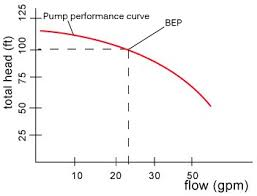## How to Calculate and Solve for Rated Static Head (in Top Gating) | Design of Gating SystemThe image represents rated static head (in top gating).

To compute for rated static head (int top gating), one essential parameter is needed and the parameter is Height of the Sprue from its Top to the Point of Metal Entry into the Mould Cavity (H).

The formula for calculating rated static head (in top gating).

Hs = H

Where:

Hs = Rated Static Head (in top Gating)
H = Height of the Sprue from its Top to the Point of Metal Entry into the Mould Cavity

Let’s solve an example;
Find the rated static head (in top gating) when the height of the sprue from its top to the point of metal entry into the mould cavity is 10.

This implies that;

H = Height of the Sprue from its Top to the Point of Metal Entry into the Mould Cavity = 10

Hs = H
Hs = 10

Therefore, the rated static head (in top gating) is 10.